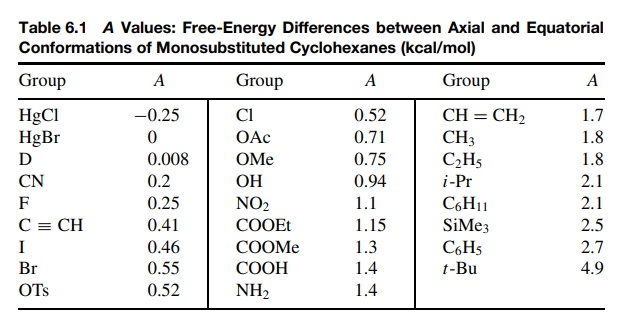# A Values

| Home | | Organic Chemistry |

## Chapter: Organic Chemistry : Stereochemical and Conformational Isomerism

Viewed another way, if the axial– equatorial energy difference is mainly a func-tion of steric bulk, then it might be used to assess the relative size of various groups.

A VALUES

Viewed another way, if the axial– equatorial energy difference is mainly a func-tion of steric bulk, then it might be used to assess the relative size of various groups. That is, if the energy difference between the two chair conformational isomers of a monosubstituted cyclohexane were measured, it might serve as a quantitative measure of the effective steric bulk of a particular group.Table 6.1 is a collection of such data. The free-energy differences between equatorial and axial substituents on a cyclohexane ring are called A values and are a quantitative measure in kilocalories per mole of the effective steric bulk of a substituent. An important point is that these A values are not a measure of the physical size of a group, but rather are a measure of its steric interactions. Thus the t -butyl group (A = 4.9) is seen to be significantly more bulky than the trimethylsilyl group (A = 2.5), yet physically the trimethylsilyl group occupies more volume. The difference is that the carbon – silicon bond is longer than the carbon – carbon bond so that the trimethylsilyl group is farther away from the ring. Thus its effec-tive bulk, which is the strength of 1,3 diaxial interactions, is actually less than the t -butyl group. The same trend is seen in the halides, where the A values for chloride (A = 0.52), bromide (A = 0.55), and iodide (A = 0.46) decrease even though the size of these atoms increase: Cl < Br < I. Thus A values are related to the effective steric bulk and not the actual physical size of substituents. In this respect they are very useful since it is clearly the effective size of substituents which gives rise to steric effects in chemical reactions. Thus A values can be used to predict steric changes resulting from the introduction of a group into a molecule. While the effective steric bulk of a group in a different molecule may not be quite the same as when it is attached to the cyclohexane ring, the trends should be parallel. In this way A values provide a useful way to evaluate steric effects semiquantitatively.